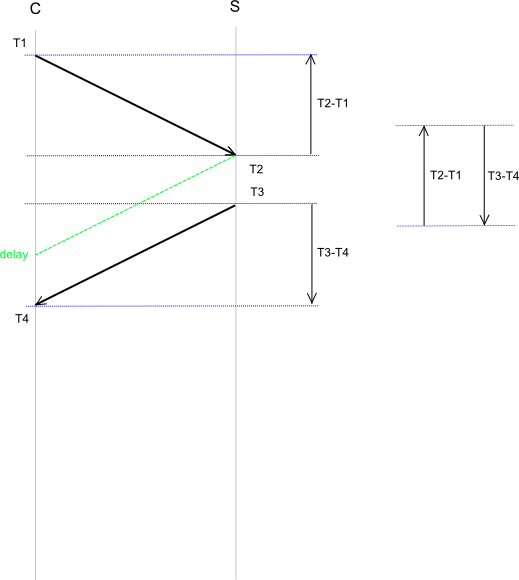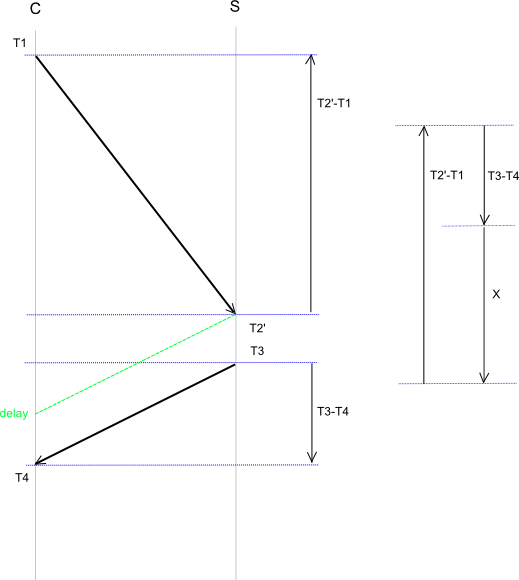Here I want to show some theoretical aspects using NTP over a network connection which has different delays in both directions. This is typical given with ADSL. Before continuing reading here you should read my first blog about NTP and ADSL.

## Symmetric situation

First lets look at a situation where delay from server to client is identical to the delay from client to server. This is also the situation where NTP works correct. For a detailed description see NTP Timestamp Calculations.

Let’s assume both time server are in sync ( have the same time )As we can easily see delay between T1 and T2 is identical with delay between T3 and T4. With the formula for offset

offset = [(T2 - T1) + (T3 - T4)] / 2

we get the result 0.

## Non symmetrical situation

Now the non symmetrical situation as it is given with ADSL for example. This ntp frame reaches the server later based on the lower upload rate in opposite to the higher download speed. We can say

T2’ = T2 + x

And “x” is the additional delay given by asymmetry.The formula for offset is now

0 = [(T2 + x - T1) + (T3 - T4)] / 2

We can set this value to 0 as we know both time server are in sync. Rewriting the mathematical formula we get

x = T1 - T2 - T3 + T4

I tracked the packet with “tcpdump” running “ntpdate -d -d -d -d -u ip.address” and got the following values:

```T1=0.410634 ; T2=0.415284 ; T3=0.415471 ; T4=0.430596
```

Of course I dropped the values left of the decimal point. The values for timestamp 2 and 3 I read out from the answer packet coming from the server. The values for timestamp 1 and 4 I took from “wireshark”. I know this is not exactly but it is also not sooo bad.

Doing the math to verify the results I got

```# echo -n "delay " ; dc <<< "7k \$T4 \$T1 -  \$T3 \$T2 - - p"
delay .019775
```

The value for delay coming from “ntpq -p” is 19.664 - so quite near my hand measured result.

```# echo -n "offset "  ; dc <<< "7k \$T2 \$T1 - \$T3 \$T4 - + 2/p "
offset -.0052375
```

Also offset fits well. -5.342 is the value from ntpq.

Now calculating x:

```# echo -n "x = "  ; dc <<< "7k \$T1 \$T2 - \$T3 - \$T4 +p"
x = .010475
```

Splitting x into two parts each 50% we have the symmetrical situation. And of course ‘ x / 2 = offset ‘ respectively ‘ x = 2 * offset ‘. This is not really surprising as the formula for “x” is coming out of the formula for offset. It is quite similar. Some signs are different and we lost factor 2. But it is also what we have seen in my previous blog.

So we know the additional delay is twice the offset we see with “ntpq” for a 90 byte UDP packet. But even with a symmetric connection to the internet there are a lot of components which produces different delays from A to B and from B to A. Therefore we never know how accurate our time information is.